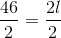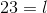ISEE Middle Level Quantitative : Squares

Example Questions

The sum of the lengths of three sides of a square is one foot. Give the perimeter of the square in inches.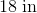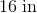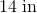It is impossible to determine the perimeter from the information given.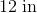Explanation:

A square has four sides of the same length.

One foot is equal to twelve inches; since the sum of the lengths of three of the congruent sides is twelve inches, each side measures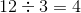inches.

The perimeter is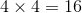inches.

A square has perimeter one yard. Which is the greater quantity?

(A) The length of one side of the square

(B) 8 inches

(A) and (B) are equal

It is impossible to determine which is greater from the information given

(A) is greater

(B) is greater

(A) is greater

Explanation:

One yard is equal to 36 inches. A square has four sides of equal length, so one side of the square has length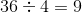inches.

Since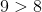, (A) is greater.

Example Question #1 : How To Find Perimeter

A square has perimeter five meters. Which is the greater quantity?

(A) 1,250 millimeters

(B) The length of one side of the square

(A) is greater

(B) is greater

(A) and (B) are equal

It is impossible to determine which is greater from the information given

(A) and (B) are equal

Explanation:

One meter is equal to 1,000 millimeters, so the square has perimeter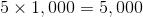millimeters.

A square has four sides of equal length, so one side of the square has length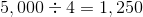millimeters.

The quantities are equal.

Example Question #11 : Squares

A square lot has perimeter one mile. Which is the greater quantity?

(A) 1,320 feet

(B) The length of one side of the square

(A) and (B) are equal

(A) is greater

It is impossible to tell which is greater from the information given

(B) is greater

(A) and (B) are equal

Explanation:

One mile is equal to 5,280 feet. A square has four sides of equal length, so one side of the square has length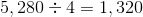feet.

The quantities are equal.

Example Question #1 : How To Find Perimeter

The sum of the lengths of three sides of a square is 9,000 centimeters. Give its perimeter in meters.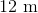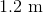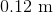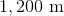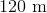Explanation:

100 centimeters are equal to one meter, so 9,000 centimeters are equal to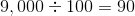meters.

A square has four sides of the same length. Since the sum of the lengths of three of the congruent sides is 9,000 centimeters, or 90 meters, each side measures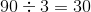meters.

The perimeter is four times this, which is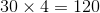meters.

A square has perimeter one meter. Which is the greater quantity?

(A) 250 centimeters

(B) The length of one side of the square

It is impossible to tell which is greater from the information given

(A) and (B) are equal

(A) is greater

(B) is greater

(A) is greater

Explanation:

One meter is equal to 100 centimeters.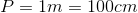A square has four sides of equal length, so we will need to divide by 4 to find the length of one side.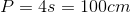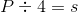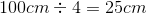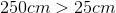, so (A) is greater

Each side of a square is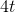units long. Which is the greater quantity?

(A)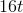(B) The perimeter of the square

(B) is greater

(A) and (B) are equal

It is impossible to determine which is greater from the information given

(A) is greater

(A) and (B) are equal

Explanation:

The perimeter of a square is four times its side length: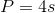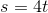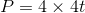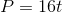Since the perimeter is equal to, (A) and (B) are equal.

Hexagon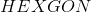and Square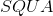both have perimeter 64. Which is the greater quantity?

(a)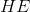(b)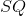It is impossible to determine which is greater from the information given

(a) and (b) are equal

(b) is the greater quantity

(a) is the greater quantity

It is impossible to determine which is greater from the information given

Explanation:

The length of one side of the square - in particular, that of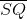- is equal to one fourth its perimeter, so it can be determined that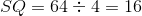. However, no relation among the sides of the hexagon is given - in particular, it is not given that the hexagon is regular - so the length of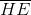cannot be determined. Insufficient information is given.

Example Question #23 : How To Find The Perimeter Of A Rectangle

What is the length of a rectangular room with a perimeter of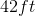and a width of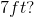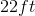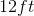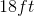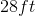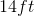Explanation: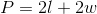We have the perimeter and the width, so we can plug those values into our equation and solve for our unknown.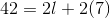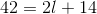Subtractfrom both sides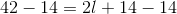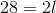Divideby both sides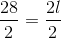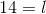Example Question #24 : How To Find The Area Of A Parallelogram

What is the length of a rectangular room with a perimeter of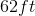and a width of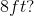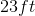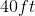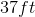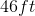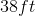Explanation:We have the perimeter and the width, so we can plug those values into our equation and solve for our unknown.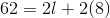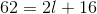Subtractfrom both sides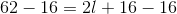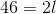Divideby both sides